# Chapter 8: Inferential statistics: going beyond the data

Quizzes are available to test your understanding of the key concepts covered in each chapter. Click on the quiz below to get started.

1. What does a significant test statistic tell us?

1. There is an important effect.
2. That the test statistic is larger than we would expect if there were no effect in the population.
3. The null hypothesis is false.
4. All of the above.

The correct answer is B. That the test statistic is larger than we would expect if there were no effect in the population.

2. What is the relationship between sample size and the standard error of the mean? (Hint: The law of large numbers applies here: the larger the sample is, the better it will reflect that particular population.)

1. The standard error decreases as the sample size decreases.
2. The standard error is unaffected by the sample size.
3. The standard error increases as the sample size increases.
4. The standard error decreases as the sample size increases.

The correct answer is D The standard error decreases as the sample size increases. This is correct because the standard error (which is the standard deviation of the distribution of sample means), defined as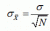, decreases as the sample size (N) increases and vice versa.

3. What symbol is used to represent the standard error of the mean?

1.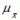2.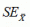3.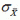4.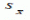4. Which of the following statements is true?

1. The standard error is calculated solely from sample attributes.
2. The standard deviation is calculated only from sample attributes.
3. The standard error is a measure of central tendency.
4. All of the above.

The correct answer is A. The standard error is calculated solely from sample attributes. This is because the standard error can be computed from a knowledge of sample attributes – sample size and sample statistics.

5. There are basically two types of statistics – descriptive and inferential. Which of the following sentences are true about descriptive statistics? (Hint: The answer is in the name descriptive statistics).

1. Descriptive statistics enable you to make decisions about your data, for example, is one group mean significantly different from the population mean?
2. Descriptive statistics describe the data.
3. Descriptive statistics enable you to draw inferences about your data, for example, does one variable predict another variable?
4. All of the above.

The correct answer is B. Descriptive statistics describe the data. Some examples are the mean and standard deviation.